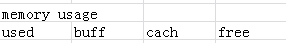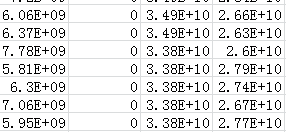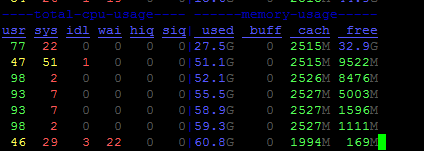# Why pytorch needs so much memory to execute distributed training?

Today I want to do distributed training in many nodes, however the memory decrease rapidly.I execute the command 'dstat -c -m '.We can see the available memory decrease from 64GB to 177MB.

My batch_size is 1 and datasets include 4000 samples,every sample is about 16MB.I use the gloo as the backend.

``````class HDF5Dataset(data.Dataset):
"""Represents an abstract HDF5 dataset.

Input params:
file_path: Path to the folder containing the dataset (one or multiple HDF5 files).
recursive: If True, searches for h5 files in subdirectories.
transform: PyTorch transform to apply to every data instance (default=None).
divide: use only 1//divide dataset
"""
def __init__(self, file_path, recursive, transform=None, divide =1):
super().__init__()
self.data_info = []
self.data_cache = {} #dict
self.transform = transform
self.length = 0
self.divide = divide

# Search for all h5 files
p = Path(file_path)
assert(p.is_dir())

if recursive:
files = sorted(p.glob('**/*.hdf5'))
else:
files = sorted(p.glob('*.hdf5'))
if len(files) < 1:
raise RuntimeError('No hdf5 datasets found')

self.length = len(files) // divide
self.data_info = files[0: self.length]

#print("File Prepared !\n")

def __getitem__(self, index):
# get data
y, x = self.get_data(index)

if self.transform:
x = self.transform(x)
else:
x = torch.from_numpy(x)
x = torch.reshape(x, (1,128,128,128))
x = x.type(torch.FloatTensor)

# get label
y = torch.from_numpy(y)
y = y.type(torch.FloatTensor)
return (x, y)

def get_data(self, i):
fp = self.data_info[i] #list - dict
#print(fp)

try:
with h5py.File(fp,'r') as h5_file:

label = h5_file.get('label')[()]
dataset = h5_file.get('dataset')[()]

return (label, dataset)
except IOError:
print('Error:File {filepath} is broken.'.format(filepath=fp))
i=i+1
return self.get_data(i)

def __len__(self):
return self.length
``````

I want to know why pytorch DDP training need more memory with the nodes expand. And how to reduce memory usage？

What is the `world_size` in your application? Each process will have its own model replica, so the total memory consumption is expected to be larger than `world_size` X (non-DDP memory footprint). Besides, DDP also creates buffers for communication, which will contribute another ~1.5X if not considering optimizer.

Thanks for your answer.I set the parameter nproc_per_node to 1 and I guess the world_size is 1.There is only one process per node.However, it still consumes a lot of memory. DDP adopts the data parallelism, I think when I train this model in more nodes, there is little memory to be consumed.
I found that the node cache always uses more than 20GB of memory and if I can not reduce the memory usage,I should choose another framework to do distributed training = = !

Hey @khalil

My batch_size is 1 and datasets include 4000 samples,every sample is about 16MB.

Does this actually mean the memory is consumed by the data loader? Can you check the memory consumption when using the same model and same data loader without DDP?

cc @vincentqb @albanD for dataloder questions

Thank you,@mrshenli. I have trained this model in one node and it consumed 30GB on the cache.Just like this，.I guess there are so many data to be loaded.Could you help me check my data_loader code?

Hey @khalil

I am trying to identify which component hogs memory. Could you please share some more details about the sentence below.

I have trained this model in one node and it consumed 30GB on the cache

By “trained this model in one node”, do you still use DDP and the same data loader? It will be helpful to know the memory footprint of the following cases:

1. Train a local model without data loader and without DDP. Just feed the model with some randomly generated tensors.
2. Train a local model with data loader but without DDP.
3. Wrap the model with DDP, and train it with data loader.

Thank you,@mrshenli,I will try it.

1 Like

I have created a small dataset which only contains 30 samples.However the memory still decreased rapidly.(batch_size=1)So I think this issue is not caused by dataloader and I am sure reason is the node expansion.

When I do DDP training in one node,the memory problem is not serious.When I expand the nodes,the memory problem is serious.I want to know if this is pytorch’s problem?

My model like this

Hey @khalil

Could you please provide details of the following question?

1. Which version of PyTorch are you using?

2. What’s the size of your model (how many GB)? You can get this by doing the following:

``````model_size = 0
for p in model.parameters():
model_size += p.numel() * p.element_size()
``````

Given the picture shown in the previous post, it looks like the model is about 27GB? If that is the case, then, yes, DDP would use another 27GB as comm buffers. We are working on improving this: https://github.com/pytorch/pytorch/issues/39022

I am curious how did you train this model locally without DDP? If the model is 27GB, after the backward pass, the grads will also consume 27GB. So local training without DDP will also use >54GB memory?

And if your optimizer uses any momentum etc., it’s likely the optimizer will also consume a few more X of 27GB. But looks like there are only 64GB memory available? Is it because your optimizer does not contain any states?

3. Does this getting worse when you use more nodes (e.g., scale from 2 nodes to 3 nodes)?

@mrshenli,Thanks for your general help.The details are as follows:

1. The version of PyTorch is 1.5.0

2. I use your code to test the size of my model and it printed this sentence: model_size is 9865484.So I think the size of model are 10MB…

3. When I scaled from 2 nodes to 4 nodes,the available memory started to decrease.The memory usage went up with the number of nodes increased.

I use your code to test the size of my model and it printed this sentence: model_size is 9865484.So I think the size of model are 10MB

In this case DDP should only consume 10MB more memory for communication buffers.

BTW, as the model is just 10MB, do you know why even without DDP it consumes 30GB? And have you seen OOM errors?

When I scaled from 2 nodes to 4 nodes, the available memory started to decrease.The memory usage went up with the number of nodes increased.

This is also not expected with DDP. DDP’s memory footprint should be constant, regardless of how many nodes are used.

Do you have a minimum repro that we can investigate?

Dear @mrshenli,forgive me for not being clear,I said it consumes 30GB which means the cache
consumes 30GB.I guess the memory is used to load the disk data.I tried to use a small datasets
which only contains 40 samples(Every sample is 16MB).And in this case,the cache consumption is about 2.5GB.However,the memory problem still exist.You can see this picture:Now I think the problem is not caused by dataloader.

And you say DDP’s memory footprint should be constant,regardless of how many nodes are used.I test the maximum memory footprint as the nodes expand,it just like this

The memory footprint does not include the cache consumption.

And when I test the memory footprint about 32 nodes,I found a memory allocated error which indicates the memory has run out.

Thanks for the detailed information. This is weird. This is the first time that we saw the memory footprint per machine increases significantly with the total number of machines.

I guess the memory is used to load the disk data.I tried to use a small datasets
which only contains 40 samples(Every sample is 16MB).And in this case,the cache consumption is about 2.5GB.

Q1: This does not add up. The model is 10MB, and the dataset is 40X16MB = 640MB. I assume you do not use CUDA, as you mainly concerned about CPU memory. In this case, I would assume the total memory consumption to be less than 1GB. Do you know where does the other 1.5GB come from?

Q2: Can you try to the following to see if it is indeed used by the process instead of some os cache.

``````import psutil

process = psutil.Process(os.getpid())

for _ in get_batch():
....
# print this in every iteration
``````

Q3: Do you have a minimum reprodueable example that we can investigate locally?

Q4: BTW, how do you launch your distributed training job? Are you using `torch.distributed.launch`. If so, could you please share the command you are using?

@mrshenli,Sorry,I can not reply you in time.

Now I found the other 1.5GB comes from system.There is 1.5GB consumption before I start this job so I think cache consumption is right.

I use the psutil module to test the process memory footprint.The result just like the following photo:

(1 node)

(16 node)

So the process memory footprint per machine does not increase with the total number of machines.However,the total memory footprint per machine increases significantly.

Then I found the process numbers increase significantly with the number of machines.I set nproc_per_node to 1 so there should be one process in every machine.However,it is not.
There is my command:
bash.sh

``````MIP=\$ip
MPORT=\$port
NPROC_PER_NODE=1
HOSTLIST=\$hostlist
COMMAND=\$HOME/sample.py --epochs=120 --workers=0 --batch-size=1 --print-freq=50 --data=\$HOME/datasets/v3
RANK=0
for node in \$HOSTLIST; do
echo \$node
ssh -q \$node \
python -m torch.distributed.launch \
--nproc_per_node=\$NPROC_PER_NODE \
--nnodes=\$SLURM_JOB_NUM_NODES \
--node_rank=\$RANK \
\$COMMAND > "log_v1_"\${SLURM_JOB_ID}"_"\${RANK}".out" &
RANK=\$((RANK+1))
done
``````

sample.py

``````....
if __name__ == '__main__':
if torch.distributed.is_available():
dist.init_process_group(backend='gloo',init_method='env://',timeout=datetime.timedelta(seconds=600))
main()
``````

In addition,I do not use CUDA and I train this model in CPUs.

1 Like

I do not think pytorch is the cause of the mistake.I will check my shell script,thank you @mrshenli

1 Like

Hi @khalil, have you solve this problem?
I am actually meeting exactly the same problem as you.

Sorry abou the delay, I have solved this problem. The reason is that I have execute the script in same node.(ssh failed) I think you can check to see if the process has started on each node.